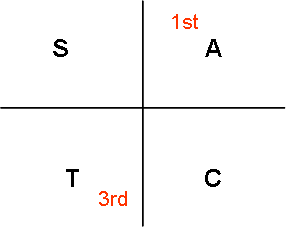## Posts

Showing posts from March, 2009

### Trigo Equations Part IISolve the following equations for  inclusive Question 1 Question 2   Question 3 What's Next ? Click here for Trigo Equations Part III Click here for Contents Page

### Trigo equations Part ISolve the following equations for Question 1 Note that when calculating the basic angle we ignore the negative sign i.e. we press 0.75 into the calculator and not -0.75 We take into consideration the sign only when determining which quadrant to use ____________________ Question 2   ____________________ The 2 questions above represent the most basic type of trigo equations. All complicated trigo equations can be reduced to the most basic type. The next post will demonstrate how to solve equations where the angles are slightly more complicated such as 2x, x/2 or 2x + 74 What's Next? Click here for Trigo Equations Part II Click here for Contents Page

### Basic TrigonometryBasic Angles / Reference angles Using your calculator, determine what is sin 30°, sin 150°, sin 210° and sin 330°? sin 30° = 0.5 sin 150° = 0.5 sin 210°= -0.5 sin 330°= -0.5 What do you think is the relationship between these angles ? 30° = 30° (1st quadrant ) 150° = 180° – 30° (2nd quadrant) 210° = 180° + 30° (3rd quadrant) 330° = 360° – 330° (4th quadrant) You would realise that sine is positive in the 1st and 2nd qudrants You can carry out the same exercise with cos and tan cosine is positive in the 1st and 4th quadrant tangent is positive in the 1st and 3rd quadrant We can use the diagram above to help us remember which functions are positive in the quadrants A means all the functions are positive in 1st quadrant T means that only tangent is positive in the 3rd quadrant I remember this as All Science Teachers are Charming Example 1 Given that for x between and Determine the value of a) sin x b) sin 2x